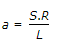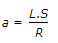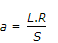# Civil Engineering - Soil Mechanics and Foundation Engineering

### Exercise :: Soil Mechanics and Foundation Engineering - Section 1

31.

Stoke's law sates that the velocity at which a grain settles out of suspension, the other factors remaining constant, is dependent upon

 A. shape of grain B. weight of grain C. size of grain D. shape and size of grain E. shape, size and weight of grain.

Explanation:

No answer description available for this question. Let us discuss.

32.

Pick up the correct statement from the following:

 A. When water table is above the base of a footing, the dry weight m should be used for soil below water table B. When water table is located somewhat below the base of a footing, the elastic wedge is partly of moist soil and partly of submerged soil, and a suitable reduction factor is used C. When water table is just at the base of the footing, no reduction factor is used D. None of these.

Explanation:

No answer description available for this question. Let us discuss.

33.

Pick up the correct statement from the following :

 A. In soils, the flow index indicates variation in shear strength with water content B. Liquid limit minus plastic limit, is known as plasticity index of the soil C. Plastic limit minus shrinkage limit, is known as shrinkage index of the soil D. The ratio of the plasticity index to the flow limit, is known as toughness index of the soil E. All the above.

Explanation:

No answer description available for this question. Let us discuss.

34.

If S, L and R are the arc length, long chord and radius of the sliding circle then the perpendicular distance of the line of the resultant cohesive force, is given by

 A.B.C.D. none of these.

Explanation:

No answer description available for this question. Let us discuss.

35.

The liquid limit and plastic limit exist in

 A. sandy soils B. silty soils C. gravel soils D. clay soils.